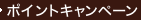•### Finite Fields (Encyclopedia of Math and its Applications) （2 SUB）

• 提携先の海外書籍取次会社に在庫がございます。通常2週間で発送いたします。
重要ご説明事項
1. 納期遅延や、ご入手不能となる場合が若干ございます。
2. 複数冊ご注文の場合、分割発送となる場合がございます。
3. 美品のご指定は承りかねます。
• ≪洋書のご注文につきまして≫ 「海外取次在庫あり」および「国内仕入れ先からお取り寄せいたします」表示の商品でも、納期の目安期間内にお届けできないことがございます。あらかじめご了承ください。

• 製本 Hardcover:ハードカバー版／ページ数 755 p.
• 言語 ENG
• 商品コード 9780521392310
• DDC分類 512.3

### Full Description

The theory of finite fields is a branch of algebra that has come to the fore because of its diverse applications in such areas as combinatorics, coding theory and the mathematical study of switching ciruits. This book is devoted entirely to the theory of finite fields, and it provides comprehensive coverage of the literature. Bibliographical notes at the end of each chapter give an historical survey of the development of the subject. Worked-out examples and lists of exercises found throughout the book make it useful as a text for advanced-level courses.

### Table of Contents

`1. Algebraic foundations2. Structure of finite fields3. Polynomials over finite fields4. Factorization of polynomials5. Exponential sums6. Equations over finite fields7. Permutation polynomials8. Linear recurring sequences9. Applications of finite fields10. Tables.`